Cel. & Mol. Biol.Let.,7, 157 - 158, 2002

SHAPE OF VESICLES IN FLOW – THEORETICAL ANALYSIS

FRANCE SEVŠEK

University College of Health Studies, University of Ljubljana, Poljanska 26A and Institute of Biophysics, Lipičeva 2 1000 Ljubljana, Slovenia

Abstract: The shapes of phospholipid vesicles in a liquid flow with a low Reynolds number were calculated within the nearly spherical approximation. The effective tension model was used in the case of large tension values.

Key Words: phospholipid vesicles, shapes, spherical harmonics

INTRODUCTION

Giant phospholipid vesicles form spontaneously in water when a thin film of amphiphilic phospholipid molecules closes in upon itself due to the hydrophobic effect. These vesicles consist of a phospholipid membrane that encloses the water solution. Their elastic properties were intensively studied, mainly as a model for biological membranes. Phospholipid membranes are virtually an incompressible and impermeable two dimensional liquid. But, as external forces dampen short wavelength shape fluctuations, the phospholipid bilayer behaves as if it were elastic. Membrane bending elasticity could in principle be determined from the measurement of thermal shape fluctuations of vesicles attached at one point to a glass plate and subjected to liquid flows of adjustable velocities. Detailed theoretical understanding of the experimental data is still lacking. Here, vesicle shape will be calculated for the high membrane tension case for axially symmetric shapes.

CALCUALTION OF THE VESICLE SHAPE

The shapes of those vesicles with shapes that are close to spherical can be conveniently expressed in terms of normalised spherical harmonics (YLM(q,f)):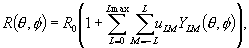where R is the distance from the chosen origin of the coordinate system to the point on the membrane surface, and uLM are the expansion coefficients.

For every surface element the pressure balance can be written as: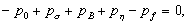where p0 is the pressure difference between inside and outside at infinity, and ps is the pressure due to the membrane surface tension. This may be related to the average membrane curvature (c) and the surface tension (s) as ps=2cs . Since for a nearly spherical vesicle the coefficients (uLM) are small, all higher order terms in the expansion of membrane curvature were neglected, yielding: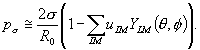The pressure due to the membrane bending rigidity (pB) will be neglected in the case of high membrane tension. A viscous liquid moving with velocity v exerts pressure on the membrane, approximated as ph=-( hv/R0)(3 p)1/2Y10 for the nearly spherical case. The pressure (pf) exerted on the membrane by the applied external force is expressed in terms of spherical harmonics, where the coefficients (pfL) will be taken to be of the form exp(-sL2/4).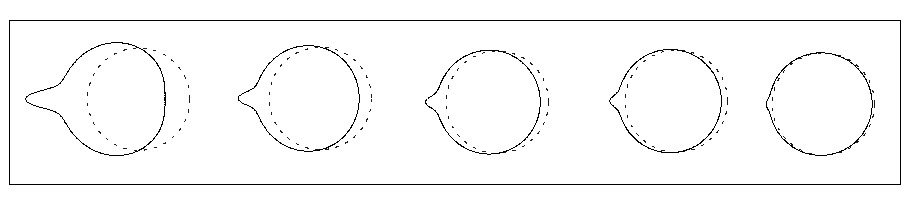Fig.1 – Vesicle shapes in flow at different membrane tensions: s=0.15, 0.31, 0.46, 0.61 and 1.5hv, increasing from left to right. The flow direction is to the right. The dashed circles correspond to the spherical shape with R0. The figures were not scaled to account for constant vesicle volume.

RESULTS AND DISCUSSION

With the above approximations, one gets for the axially symmetric case: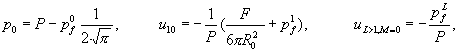where Pş2s/R0 and F is the Stokes force (F=6pR0hv).

As bending energy was neglected and the viscous pressure (ph) for nearly spherical shapes depends only on L=1, we see that the shape is mainly determined by the external force. This is illustrated in Fig.1 where the vesicle shapes were calculated for some different values of membrane tension.

REFERENCES

1. Sevšek, F. Gomišček, G., Arrigler, V., Svetina, S. and Žekš, B., Thermal fluctuations of tethered vesicles, Abstracts, (Journal of Biosciences, Vol. 24, Suppl. 1), Bangalore: Indian Academy of Sciences, 113, 1999
2. Landau L.D and Lifshitz E.M., Fluid Mechanics, 2nd edition, Butterworth-Heinemann Ltd, Oxford 1987

Cel. & Mol. Biol.Let.,7, 157 - 158, 2002